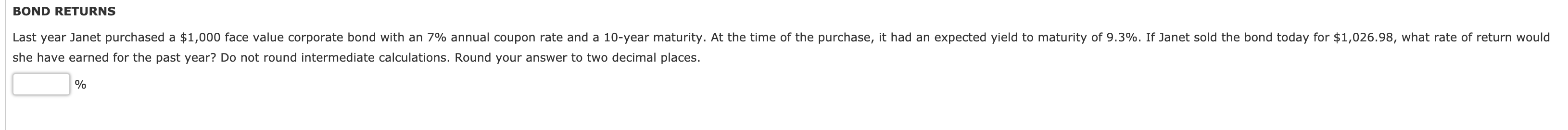Question

# 25-year bond has a \$1,000 face value, a 10% yield to maturity, and an 8% annual...

25-year bond has a \$1,000 face value, a 10% yield to maturity, and an 8% annual coupon rate, paid semi-annually. What is the market value of the bond?

Suppose a bond with a 10% coupon rate and semiannual coupons, has a face value of \$1000, 20 years to maturity and is selling for \$1197.93. What’s the YTM?

Face value = \$1,000

Annual coupon rate = 8%
Semiannual coupon rate = 4%
Semiannual coupon = 4% * \$1,000
Semiannual coupon = \$40

Annual YTM = 10%
Semiannual YTM = 5%

Time to maturity = 25 years
Semiannual period = 50

Current price = \$40 * PVIFA(5%, 50) + \$1,000 * PVIF(5%, 50)
Current price = \$40 * (1 - (1/1.05)^50) / 0.05 + \$1,000 / 1.05^50
Current price = \$817.44

#### Earn Coins

Coins can be redeemed for fabulous gifts.

Similar Homework Help Questions
• ### 1) Consider a 10-year bond trading at \$1150 today. The bond has a face value of...

1) Consider a 10-year bond trading at \$1150 today. The bond has a face value of \$1,000, and has a coupon rate of 8%. Coupons are paid semiannually, and the next coupon payment is exactly 6 months from now. What is the bond's yield to maturity? 2)A coupon-paying bond is trading below par. How does the bond's YTM compare to its coupon rate? a. Need more info b. YTM = Coupon Rate c. YTM > Coupon Rate d. YTM <...

• ### Q4 - Bond Valuation (25 min) Value the following bonds 20-year bond with a face value...

Q4 - Bond Valuation (25 min) Value the following bonds 20-year bond with a face value of \$10,000 with an annual coupon of 5% and market rate (yield to maturity or YTM) of 6.5% 10-year bond with a coupon of 8% (split into quarterly payments), face value of \$5000 and YTM of 7% (annually) 5-year bond with a face value of \$4,000, with semi-annual coupon payments, with a coupon rate equal to YTM.

• ### Tyson Corporation has an outstanding issue of 25-year maturity corporate bond with face value of \$1,000...

Tyson Corporation has an outstanding issue of 25-year maturity corporate bond with face value of \$1,000 and a coupon of 4%, paying coupon interest semi-annually. If the market rate of interest (YTM) is 6% on similar risk bonds, at what price would this bond trade in the market.

• ### You currently own a 25-year maturity Government of Canada bond with a face value of \$1000...

You currently own a 25-year maturity Government of Canada bond with a face value of \$1000 that was issued Oct 15, 2015 (i.e. 5 years ago) with a 6% coupon paid semi-annually. The current price of the bond is \$1075. a) What is the current YTM of this Government of Canada bond? Assume semi-annual compounding. b) You also own a Corporate bond that will mature in 20 years. It also pays a semi-annual coupon of 6% and has a face...

• ### BOND RETURNS Last year Janet purchased a \$1,000 face value corporate bond with an 7% annual...BOND RETURNS Last year Janet purchased a \$1,000 face value corporate bond with an 7% annual coupon rate and a 10-year maturity. At the time of the purchase, it had an expected yield to maturity of 9.3%. If Janet sold the bond today for \$1,026.98, what rate of return would she have earned for the past year? Do not round intermediate calculations. Round your answer to two decimal places. % BOND VALUATION Madsen Motors's bonds have 12 years remaining to...

• ### A bond that compounds annually has a Face Value of \$1,000 and maturity of 15 years....

A bond that compounds annually has a Face Value of \$1,000 and maturity of 15 years. Assume that its coupon rate is 8% and yield to maturity (YTM) is 6.12%. What is this bond’s market price?

• ### 1.) Suppose a five-year, \$1,000 bond with annual coupons has a price of \$900.00 a...continues

1.) Suppose a five-year, \$1,000 bond with annual coupons has a price of \$900.00 and a yield to maturity of 6.0%. What is the bond's coupon rate?2.) The yield to maturity of a 2 year \$1,000 bond with a 8.5% coupon rate and semiannual coupons is 9.1% APR, compounded semi-annually. What must its price be?

• ### The 10-year Coupon Bond has a face value of \$1,000, the annual coupon rate is 5...

The 10-year Coupon Bond has a face value of \$1,000, the annual coupon rate is 5 percent (out of its face value), the yield to maturity is 10 percent. (2.a) show me the cash flows of this coupon bond, you can use words or a timeline graph you created. (2.b) compute the price (present value) of this bond (2.c) suppose the yield to maturity increases to 20 percent after one year, computes the new price. (remember that as time passed...

• ### If a bond with a face value of \$1,000, 8 years to maturity, semiannual coupons, and...

If a bond with a face value of \$1,000, 8 years to maturity, semiannual coupons, and the yield to maturity of 4% sells for \$1,067.89, what is its annual coupon rate? A. 4% B. 5% C. 2% D. 3% E. 2.5%

• ### A.Zero Coupon Bonds A 7 year maturity zero coupon corporate bond has an 8% promised yield....

A.Zero Coupon Bonds A 7 year maturity zero coupon corporate bond has an 8% promised yield. The bond's price should equal B.The Fishing Pier has 6.40 percent, semi-annual bonds outstanding that mature in 12 years. The bonds have a face value of \$1,000 and a market value of \$1,027. What is the yield to maturity? C.Bond Yields Find the promised yield to maturity for a 7% coupon, \$1,000 par 20 year bond selling at \$1115.00. The bond makes semiannual coupon...